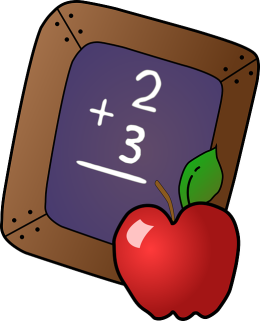Marvelous MathOPERATIONS AND ALGEBRAIC THINKING

• Write and interpret numerical expressions using parentheses, brackets, braces; generate number patterns using rules; form ordered pairs

NUMBERS AND OPERATIONS IN BASE TEN

• Understand the place value system; perform operations (add, subtract, multiply, divide) with multi-digit whole numbers and with decimals to the hundredths

NUMBERS AND OPERATIONS-FRACTIONS

• Add, subtract, multiply, and divide fractions and mixed numbers with like and unlike denominators; interpret fractions as division

MEASUREMENT, DATA, GEOMETRY

• Convert among different-sized measurement units; represent and interpret date on line plots; understand concepts of volume; graph points on a coordinate plane; classify two-dimensional figures into categories

FloridaStudents.org - excellent tutorials

Studyjams - fun! and educational

Mr. Math Blog - step by step lesson tutorials

Create A Graph

Multiplication Rock - songs for the times tables

Multiplication Website

Kids Numbers

Online Math Learning

Helping With Math - various subject areas

A Plus Math - flashcard practice

Beat the Clock game - telling time practice

Kids Numbers - excellent learning games

Shape Surveyor - area & perimeter game

Money Maker - money game

Place Value Puzzler - place value game

Math is Fun - practice games

Super Kids - worksheets

Rick's Math - worksheets

It's not unusual for children to have trouble learning the multiplication facts. Fortunately, you can help if you're willing to work with your child every day. For the best results, keep drill sessions short, review learned facts frequently, and don't teach a new set of facts until your child has completely memorized the previous set. Begin by doing the following:

1. Make a set of multiplication flash cards with your child. Do not include the answers on the cards.

2. Work with one set of facts at a time (2x1, 2x2, 2x3, 2x4, etc.)

3. Next, work with the set of facts that has 2 as a second factor (1x2, 2x2, 3x2, 4x2, etc.) Remind your child that these facts are equivalent to addition doubles.

4. In the next session, work with the 5x tables. Start with 5 as the first factor (5x1, 5x2, 5x3) and then tackle 5 as the second factor (1x5, 2x5, 3x5).

5. For some children, it helps to recognize patterns when then exist within each set of facts.

6. To help your child with the 4x tables, you can teach the double and then double again approach. For example, 4x3=12 because double 3 is 6 and double again is 12; 4x4=16 because double of 4 is 8 and double again is 16, and so on.

7. To help your child with the 9x tables, teach the -1 approach. For example, 2x9=18 because 2-1 is 1 and 9-1 is 8; put them together and you get 18. Similarly, 3x9=27 because 3-1 is 2 and 9-2 is 7; put them together and you get 27.

When your child doesn't know a fact, don't tell the answer. Answers that come easily are not retained. Instead, show how to find the answer. For example, to figure out 3x4 the child can draw 3 parallel horizontal lines and 4 parallel vertical lines. Then count the intersections to find the answer.